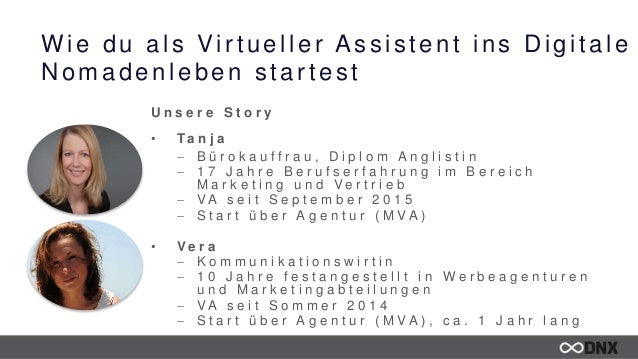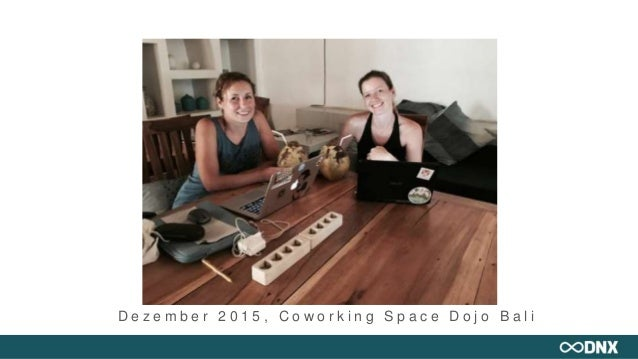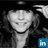Successfully reported this slideshow.Upcoming SlideShare
×

ofUpcoming SlideShare
DNX Workshop ★ Facebook Ads - So erreichst du mit wenig Geld und Aufwand genau deine Zielgruppe - Sarah Lorenz
Next

Share

# DNX Workshop ★ Wie du als Virtueller Assistent ins Digitale Nomadenleben startest - Vera Ruttkowski & Tanja Baumann

www.dnx-berlin.de > DNX ★ Digitale Nomaden Konferenz

See all

See all

### DNX Workshop ★ Wie du als Virtueller Assistent ins Digitale Nomadenleben startest - Vera Ruttkowski & Tanja Baumann

1. 1. Wie du als Virtueller Assistent ins Digitale Nomadenleben startest U n s e r e S t o r y • T a n j a  B ü r o k a u f f r a u , D i p l o m A n g l i s t i n  1 7 J a h r e B e r u f s e r f a h r u n g i m B e r e i c h M a r k e t i n g u n d V e r t r i e b  V A s e i t S e p t e m b e r 2 0 1 5  S t a r t ü b e r A g e n t u r ( M V A ) • V e r a  K o m m u n i k a t i o n s w i r t i n  1 0 J a h r e f e s t a n g e s t e l l t i n W e r b e a g e n t u r e n u n d M a r k e t i n g a b t e i l u n g e n  V A s e i t S o m m e r 2 0 1 4  S t a r t ü b e r A g e n t u r ( M V A ) , c a . 1 J a h r l a n g
2. 2. D e z e m b e r 2 0 1 5 , C o w o r k i n g S p a c e D o j o B a l i
3. 3. Was macht ein Virtueller Assistent? • S e h r v i e l f ä l t i g e A u f g a b e n b e r e i c h e • A d m i n i s t r a t i v e U n t e r s t ü t z u n g f ü r U n t e r n e h m e r , F r e i b e r u f l e r o d e r a u c h P r i v a t p e r s o n e n • I m e n g l i s c h s p r a c h i g e n R a u m s c h o n v i e l v e r b r e i t e t e r • 1 9 9 8 g a b e s c a . 1 0 0 0 V A s , h e u t e a l l e i n i n d e n U S A c a . 1 0 . 0 0 0 V i r t u e l l e A s s i s t e n t e n . W e l t w e i t w i r d d i e A n z a h l a u f c a . 3 5 . 0 0 0 g e s c h ä t z t .
4. 4. Was macht ein Virtueller Assistent? - Beispiele • C o p y w r i t i n g • R e c h e r c h e • R e i s e p l a n u n g • S o c i a l M e d i a M a r k e t i n g • C o m m u n i t y M a n a g e m e n t • B u c h h a l t u n g • P r ä s e n t a t i o n e n • W e b s e i t e n p f l e g e n • B l o g a r t i k e l e i n s t e l l e n • V e r t r i e b • K u n d e n k o m m u n i k a t i o n • P o d c a s t s t r a n s k r i b i e r e n • K o o r d i n a t i o n v o n F r e e l a n c e r n • …
5. 5. Welche Skills brauche ich als VA? • O h n e g e w o h n t e n B ü r o a l l t a g z u a r b e i t e n k a n n s c h w i e r i g s e i n • U m s t e l l u n g a u f S e l b s t ä n d i g k e i t e r f o r d e r t e i n g u t e s Z e i t m a n a g e m e n t u n d D i s z i p l i n • A r b e i t s w e i s e d e m M e n s c h e n a n p a s s e n u n d n i c h t u m g e k e h r t
6. 6. Welche Skills brauche ich als VA? • S e l b s t d i s z i p l i n • O r g a n i s a t i o n s f ä h i g k e i t • F l e x i b i l i t ä t • B e g e i s t e r u n g s f ä h i g k e i t • F i t i n d i v e r s e n S o f t w a r e t o o l s ( M i n i m u m s o l l t e n K e n n t n i s s e i n e i n e m E - M a i l P r o g r a m m u n d W o r d s e i n ) • O f f e n n e u e s z u l e r n e n • T o p S k i l l s o B l o g M a n a g e m e n t o E - M a i l M a r k e t i n g S u p p o r t o S o c i a l M e d i a M a n a g e m e n t o K u n d e n s e r v i c e
7. 7. Wo finde ich meine Kunden? • S i c h s e l b s t z u p r o m o t e n i s t n i c h t j e d e r m a n n s S a c h e • F ü r e i n e A g e n t u r a r b e i t e n , n u r e i n m a l b e w e r b e n n o t w e n d i g . • A l t e A r b e i t g e b e r f r a g e n • I n F a c e b o o k G r u p p e n o d e r C o m m u n i t i e s b e i t r e t e n • K o n t a k t e n u t z e n
8. 8. Wo finde ich meine Kunden? • A g e n t u r e n f ü r v i r t u e l l e A s s i s t e n t e n o M e i n - v i r t u e l l e r a s s i s t e n t . c o m o S t r a n d s c h i c h t • J o b b ö r s e n • X i n g • E m p f e h l u n g e n • A l t e A r b e i t g e b e r • P o r t a l e o u p w o r k o m a c h d u d a s . d e o f i v e r r
9. 9. Welche Tools sollte ich beherrschen? • T r e l l o o B a s e c a m p , A s a n a , R e d m i n e , C o S c h e d u l e • W u n d e r l i s t • E v e r n o t e • G o o g l e D r i v e • D r o p b o x • S l a c k • S k y p e • S i p g a t e • W o r d p r e s s • F a s t B i l l • O f f i c e
10. 10. Was kann ich als VA verdienen? • O r i e n t i e r u n g a n d e n V A A g e n t u r e n ( b e i m e i n - v i r t u e l l e r a s s i s t e n t a b 1 8 , 9 0 / S t u n d e ) • P r o S t u n d e o d e r P a u s c h a l e n • P r o b e m o n a t • A b h ä n g i g v o n A u f g a b e n a r t u n d S t u n d e n a n z a h l • A G B s , V e r t r ä g e , V e r t r a u l i c h k e i t s v e r e i n b a r u n g e n
11. 11. Weitere Infos • v a - f a b r i k . d e – U n s e r O n l i n e k u r s f ü r V A ‘ s • f e r n a r b e i t . n e t – C o m m u n i t y u n d J o b b ö r s e • f r e i v o g e l . z o n e – Ta n j a s B l o g • v e r a v a . d e – V e r a s B l o g
•#### JozianeRodrigues4

Sep. 16, 2019
•#### JeannettPaulin

Feb. 8, 2017
•#### CarolaMller1

Jan. 23, 2017

Total views

1,889

On Slideshare

0

From embeds

0

Number of embeds

4

38

Shares

0